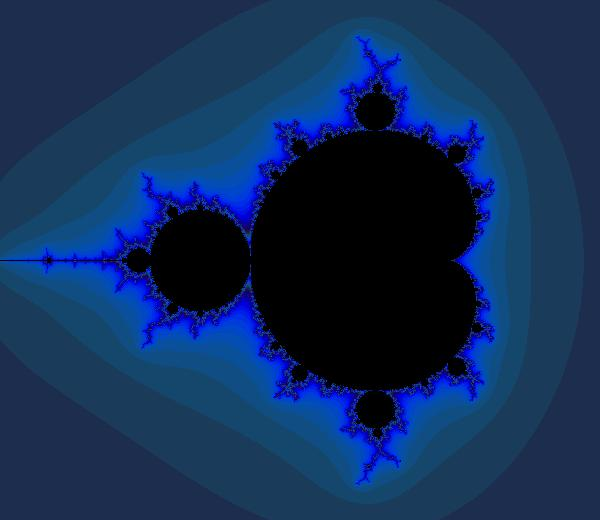# W3cubDocs

/TensorFlow Guide

# Mandelbrot Set

Visualizing the Mandelbrot set doesn't have anything to do with machine learning, but it makes for a fun example of how one can use TensorFlow for general mathematics. This is actually a pretty naive implementation of the visualization, but it makes the point. (We may end up providing a more elaborate implementation down the line to produce more truly beautiful images.)

## Basic Setup

We'll need a few imports to get started.

```# Import libraries for simulation
import tensorflow as tf
import numpy as np

# Imports for visualization
import PIL.Image
from io import BytesIO
from IPython.display import Image, display
```

Now we'll define a function to actually display the image once we have iteration counts.

```def DisplayFractal(a, fmt='jpeg'):
"""Display an array of iteration counts as a
colorful picture of a fractal."""
a_cyclic = (6.28*a/20.0).reshape(list(a.shape)+)
img = np.concatenate([10+20*np.cos(a_cyclic),
30+50*np.sin(a_cyclic),
155-80*np.cos(a_cyclic)], 2)
img[a==a.max()] = 0
a = img
a = np.uint8(np.clip(a, 0, 255))
f = BytesIO()
PIL.Image.fromarray(a).save(f, fmt)
display(Image(data=f.getvalue()))
```

## Session and Variable Initialization

For playing around like this, we often use an interactive session, but a regular session would work as well.

```sess = tf.InteractiveSession()
```

It's handy that we can freely mix NumPy and TensorFlow.

```# Use NumPy to create a 2D array of complex numbers

Y, X = np.mgrid[-1.3:1.3:0.005, -2:1:0.005]
Z = X+1j*Y
```

Now we define and initialize TensorFlow tensors.

```xs = tf.constant(Z.astype(np.complex64))
zs = tf.Variable(xs)
ns = tf.Variable(tf.zeros_like(xs, tf.float32))
```

TensorFlow requires that you explicitly initialize variables before using them.

```tf.global_variables_initializer().run()
```

## Defining and Running the Computation

Now we specify more of the computation...

```# Compute the new values of z: z^2 + x
zs_ = zs*zs + xs

# Have we diverged with this new value?
not_diverged = tf.abs(zs_) < 4

# Operation to update the zs and the iteration count.
#
# Note: We keep computing zs after they diverge! This
#       is very wasteful! There are better, if a little
#       less simple, ways to do this.
#
step = tf.group(
zs.assign(zs_),
ns.assign_add(tf.cast(not_diverged, tf.float32))
)
```

... and run it for a couple hundred steps

```for i in range(200): step.run()
```

Let's see what we've got.

```DisplayFractal(ns.eval())
```Not bad!

© 2018 The TensorFlow Authors. All rights reserved.
Licensed under the Creative Commons Attribution License 3.0.
Code samples licensed under the Apache 2.0 License.
https://www.tensorflow.org/tutorials/mandelbrot